Function Repository Resource:

# LehmerCodeFromPermutation

Create the Lehmer code corresponding to a given permutation

Contributed by: Ed Pegg Jr
 ResourceFunction["LehmerCodeFromPermutation"][perm] creates the Lehmer code corresponding to permutation perm.

## Details and Options

The generating algorithm is as follows: For each number in the permutation, count how many subsequent values it exceeds.

## Examples

### Basic Examples (1)

Generate the Lehmer code of a permutation:

 In:=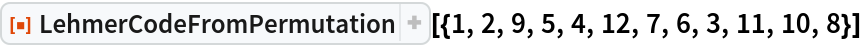Out=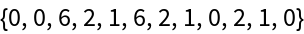### Scope (1)

Generate the Lehmer code for a particular permutation:

 In:=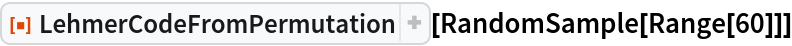Out=### Properties and Relations (2)

Multiplying by factorials leads to the factorial number system representation or lexicographic index of the given permutation:

 In:=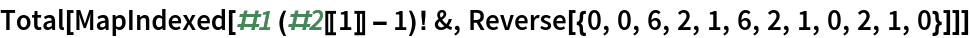Out=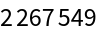The lexicographic index of a permutation works as expected:

 In:=Out=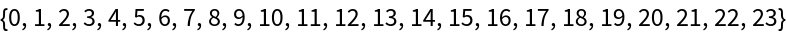## Requirements

Wolfram Language 11.3 (March 2018) or above

## Version History

• 2.0.0 – 20 December 2019
• 1.0.0 – 25 January 2019